Sie sind hier: ICP » R. Hilfer » Publikationen

# 2 Geometrical Problems in Porous Media

[374.3.1] A two-component porous sample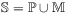is defined as the union of two closed subsets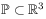and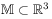where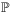denotes the pore space (or component 1 in a heterogeneous medium) and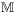denotes the matrix space (or component 2). [374.3.2] For simplicity only two-component media will be considered throughout this paper, but most concepts can be generalized to media with an arbitrary finite number of components. [374.3.3] A particular pore space configuration may be described using the characteristic (or indicator) function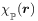of a set. [374.3.4] It is defined for arbitrary setsas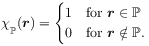(1)

[page 375, §1]    [375.1.1] The geometrical problems in porous media arise because in practice the pore space configurationis usually not known in detail. [375.1.2] On the other hand the solution of a physical boundary value problem would require detailed knowledge of the internal boundary, and hence of.

[375.2.1] While it is becoming feasible to digitize samples of several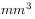with a resolution of a few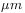this is not possible for larger samples. [375.2.2] For this reason the true pore spaceis often replaced by a geometric model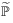. [375.2.3] One then solves the problem for the model geometry and hopes that its solution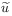obeys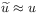in some sense. [375.2.4] Such an approach requires quantitative methods for the comparison ofand the model. [375.2.5] This in turn raises the problem of finding generally applicable quantitative geometric characterization methods that allow to evaluate the accuracy of geometric models for porous microstructues. [375.2.6] The problem of quantitative geometric characterization arises also when one asks which geometrical characteristics of the microsctructurehave the greatest influence on the properties of the solution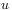of a given boundary value problem.

[375.3.1] Some authors introduce more than one geometrical model for one and the same microstructure when calculating different physical properties (e.g. diffusion and conduction). [375.3.2] It should be clear that such models make it difficult to extract reliable physical or geometrical information.